### Home > INT3 > Chapter Ch12 > Lesson 12.1.1 > Problem12-13

12-13.
1. If π < θ <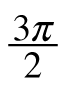, what is the sign of each expression? Homework Help ✎

1. sin(θ)

2. cos(θ)

3. tan(θ)

4.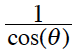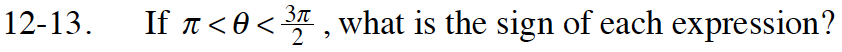You are in the third quadrant.

What are the signs of x and y in the third quadrant?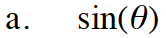The y-values are negative.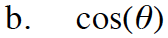Use the same method as part (a). It might also help to think about how the sin(x) and cos(x) graphs relate to each other.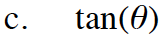Use the same method as part (a).

Negative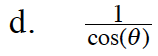Does taking the reciprocal of something change its sign?